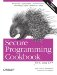# Computing the Number of Elements in a Container

Problem

You want to find the number of elements in a container.

Solution

You can compute the number of elements in a container by using the size member function or the distance function from the header as in Example 11-1.

Example 11-1. Computing the Number of Elements in a Container

```#include
#include
#include

using namespace std;

int main( ) {
vector v;
v.push_back(0);
v.push_back(1);
v.push_back(2);
cout << v.size( ) << endl;
cout << distance(v.begin( ), v.end( )) << endl;
}```

The program in Example 11-1 produces the following output:

```3
3```

Discussion

The size member function, which returns the number of elements in a standard container, is the best solution in cases where the container object is accessible. I also demonstrated distance in Example 11-1, because when writing generic code it is common to work with only a pair of iterators. When working with iterators, you often don't have access to the type of the container or to its member functions.

The distance function, like most STL algorithms, is actually a template function. Since the type of the template argument can be deduced automatically by the compiler from the function arguments, you don't have to explicitly pass it as a template parameter. You can, of course, write out the template parameter explicitly if you want to, as follows:

` cout << distance::iterator>(v.begin( ), v.end( )) << endl;`

The distance function performance depends on the kind of iterator used. It takes constant time if the input iterator is a random-access iterator; otherwise, it operates in linear time. (Iterator concepts are explained in Recipe 7.1.)

Recipe 15.1Secure Programming Cookbook for C and C++: Recipes for Cryptography, Authentication, Input Validation & More
ISBN: 0596003943
EAN: 2147483647
Year: 2006
Pages: 241